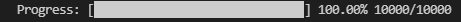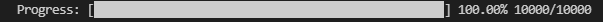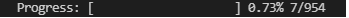#DAY 29
0
Software Development

## 自製 Progress Bar

``````class ProgressBar:
bar_string_fmt = "\rProgress: [{}{}] {:.2%} {}/{}"
cnt = 0

def __init__(self, total, bar_total=20):
self.total = total

# progress bar 的長度，可依個人喜好設定
self.bar_total = bar_total

def update(self, step=1):
# 更新 progress bar 的進度

total = self.total
self.cnt += step

# bar 的數量
bar_cnt = (int((self.cnt/total)*self.bar_total))
# 空白的數量
space_cnt = self.bar_total - bar_cnt

# 顯示 progress bar
# "\r" 的意思代表 replace，print 出來的字串不會印在新的一行而是 replace 原本那行同個位置的字符
# {:.2%}，表示 format 進來的值會以百分比顯示，並只取到小數點後兩位
progress = self.bar_string_fmt.format(
"█" * bar_cnt,
" " * space_cnt,
self.cnt/total,
self.cnt,
total
)

print(progress, end="")

percent = self.cnt/total
# 100%
if percent == 1:
print("\n")
elif percent >= 1:
print("")

if __name__ == '__main__':
# 測試
total = 10000
progress_bar = ProgressBar(total)

for _ in range(total):
# do something...
progress_bar.update()
``````bar_total = 30（靜態圖）：bar_total = 50（靜態圖）：``````
class DailyPriceSpider:

def __init__(self, progress_bar=None):

# 重複使用 TCP 連線
self.req = requests.Session()
self.url = "https://www.twse.com.tw/exchangeReport/STOCK_DAY"

# 偽裝 User-Agent
ua = UserAgent()

# 加入 progress bar 這個類別
if progress_bar:
self.progress_bar = progress_bar

# ...省略

def scrape(self, date, stock_no, save_path=""):
res = self.__get(date, stock_no)
if save_path:
res_text = res.text
self.__save_file(res_text, save_path)

# 更新 progress bar
if self.progress_bar:
self.progress_bar.update()

return res
``````

``````if __name__ == '__main__':
# ...省略

stock_info_list = stock_info_list_file.get("stock", [])
# 加入 progress bar
progress_bar = ProgressBar(len(stock_info_list))
dps = DailyPriceSpider(progress_bar=progress_bar)

# ...省略
``````

（靜態圖）